Courses

# Chapter 2 (Part 2) BJT and FET - Notes, Basic Electronics, Electrical Engineering Electrical Engineering (EE) Notes | EduRev

## Electrical Engineering (EE) : Chapter 2 (Part 2) BJT and FET - Notes, Basic Electronics, Electrical Engineering Electrical Engineering (EE) Notes | EduRev

The document Chapter 2 (Part 2) BJT and FET - Notes, Basic Electronics, Electrical Engineering Electrical Engineering (EE) Notes | EduRev is a part of the Electrical Engineering (EE) Course Electrical Engineering SSC JE (Technical).
All you need of Electrical Engineering (EE) at this link: Electrical Engineering (EE)

BJT and FET

TRANSISTOR (BJT/FET) BIASING AND STABILIZATION

The transistor is biased (with the help of external voltage in such a manner so the Q-point is selected in the middle portion the transistor output characteristic.
When the ac voltage is superimposed it is ensure that the positive and negative half of input voltage cycle remain in the linear or active region in the transistor characteristic. Under these condition the transistor output voltage is undistorted. 

• To fix the Q-point load line curve is needed. 
• The dc load line is locus of variation of output voltage and output current. 
• The load lines are being drawn for the amplifier chosen in the active region of output of characteristic. 
• The Q-point is generally fixed at the middle of load line.  Ac load line have greater slope than dc load line. 
• If the Q-point is chosen near the Vce (Cut-off region or Ic axis (saturation region) than output will be highly distorted. 
• If the Q-point is chosen in the saturation region for NPN transistor, it may cause the output to be clipped in the positive half cycle of the input signal.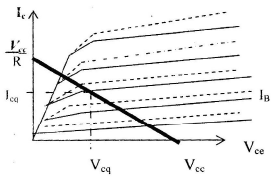Stabilization

Once the 0-point has been fixed due to some biasing arrangement it has to be stabilized against the variation in:

(a) Temperature (T) - Ico double for every 10 degree rise in temperature.
(b) IB (Depends on Vbe), i.e. Vbe decrease at the rate of 2.5 mv/degree.
(c) b 

• Due to variation of one or all of these parameter Ic current will change which shift the Q-point. 
• In stabilization technique resistive biasing circuit is used which permit Ib to vary by an amount so as to keep Ic relatively constant with variation in any of T, Ib, b

Change in Temperature 

• We Know that Ic = bIb + (1 + b) Ico  .
• b Varies due to change in temp. 
• Ib also changes due to change in temp. As the cut in voltage is increases at 2.5 mv/degree for constant Ic. 
• Ico changes with temperature doubles with every 10 degree rise in temperature. Increase in Ico caused an increase in Ic which further increasing the collector dissipation thereby increasing the collector temperature. This may set in a cumulative effect causing thermal runway burning out the transistor ultimately.

In Si transistor. Ico, is of the order of nano amperes compared to milliamps in Ge and so thermal runway and instability is more problem in Ge transistor.

Stability Factor 

the merit of a biasing circuit in holding the dc collector current Ic at the operating Q-point may be examine by stability factor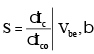= constant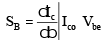= constant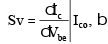= constan

•  Lower the value of stability factor (S) better is stability of circuit.

I < S < 1 + b. 

• The stability factor S is most important since it depends upon Ico and temperature.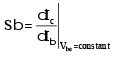IC = bIb+(1 +b)Ico....general expression Differentiate with respect to IC.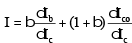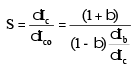• For fixed bias arrangement Ib is independent of IC, S=b+1 
• Collector to base biasing: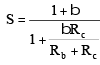a) Better than fixed biased circuit.

b) bRc >> Rb as this will give very small value as stability as near to 1.

Drawback of Collector to Base Bias 

• Rb has to be very small but this gives large Ib This increase in Ib is offset by a decrease in Ib hence there in not much overall improvement in stability. 
• Output is feedback to the base via Rh and this reduces the net gain of the transistor.

Self-Bias/Potential divider/Emitter Bias 

• AS compared to collector-base bias, if the load of the collector is a transformer, than Rc will be small bRc>> Rb cannot be achieved. But selfbias is suitable for any type of load resistance. 
• Emitter bias is applied by a resistor Rs between the emitter and ground, i.e. Thevenin equivalent of two resistor of self bias.
• When collector current increases the voltage drop across Rc increases and reduce forward bias Vbe reducing base current Ib and keeping the operating point stable 
• Stability factor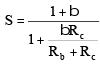• Re >>Rb,S= 1 Best stability. 
• Re introduces both dc and ac feedback. 
• In order to avoid the loss of ac signal gain because of feed back caused by Re. This Re is bypassed by a leakage capacitor Ce of high value so that is offering little resistance at frequency of interest.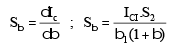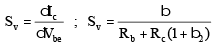where S2: Stability (S) at b = b2.
Total increment in Ic is the sum of all three increments due to Ico, b , and Vbe.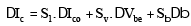Compensation Techniques 

• Major disadvantage of the resistor biasing techniques is the feed back of the output signal to input which causes reduction in gain. To avoid this, compensation technique with diodes, transistor and Thermsitor are employed. 
• .Diode compensation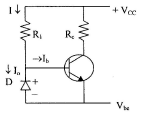• Reversed biased diode is connected in the base emitter circuit, which is same material as transistor.
•  The reverse bias voltage Vbe. allowing the reverse saturation current to Io to flow through the diode D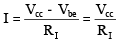And IB =I – Io
From collector current expression
Ic = b  Ib + (1 + b) Ico = b Io + (1 + b) Ico
= bI – b Io+ b ICo if b >> and Io of diode and Ico of transistor track each other over the desired temperature rang, then Ico remains essntially constant.
Reverse saturation current Io flows through the diode. If Ico increases due to temperature, the increased current flows through diode decreasing Ib keeping Ib constant.

Thermistor

Thermistors are temperature sensitive element, which has a negative temperature coefficient of resistance.
The thermistor element is placed parallel to and across Vcc and E. R2 of potential divider bias. Increase of temperature reduces resistance and decrease the forward bias reduces Ib and Ic.

Sensistor Compensation It may be placed either in parallel with R1 or parallel with RE. It can also placed in place of RE rather than in parallel with RE.
FET Biasing For the field-effect transistor, the relationship between input and output quantities is nonlinear due to the squared term in Shockley’s equation. The nonlinear relationship between ID and VGS can complicated the mathematical approach to the dc analysis of FET configurations. A graphical approach may limit solutions to tenths-place accuracy, but it is a quicker method for most FET amplifiers.
Another distinct difference between the analysis of BJT and FET transistors is that the input controlling variable for a BJT transistor is a current level, while for the FET a voltage is the controlling variable. In both cases, however, the controlled variable on the

output side is a current level that also defines the important voltage levels of the output circuit.

The general relationships that can be applied to the analysis of all FET amplifiers are IG @ OA and ID =

Is For JEETS and depletion-type MOSFETs, Shockley’s equation is applied to re-late the input and output quantities: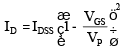For Enhancement MOSFET ID = k (VGS – Vr)2
It is particularly important to realize that of the equations above are for the device only! They do not change with each network configurations. So long as the device is in the active region.

Table FET Bias Configurations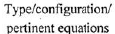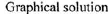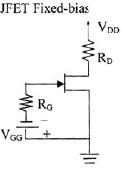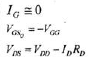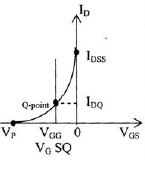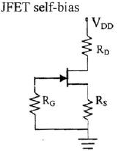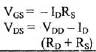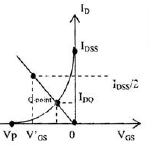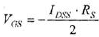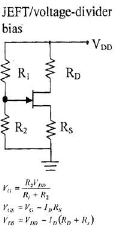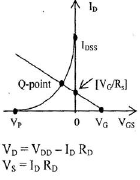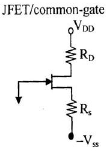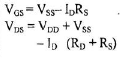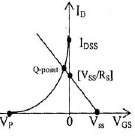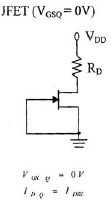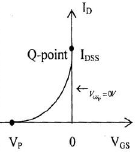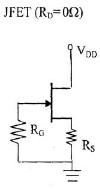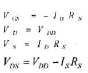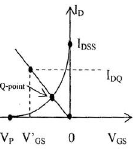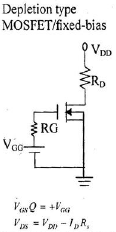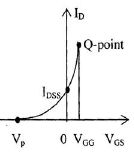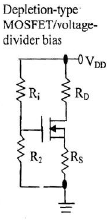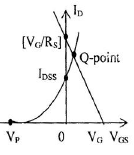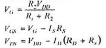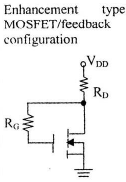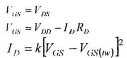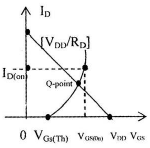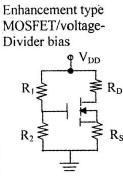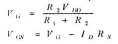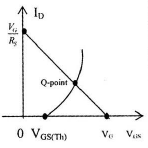Offer running on EduRev: Apply code STAYHOME200 to get INR 200 off on our premium plan EduRev Infinity!

65 docs|37 tests

,

,

,

,

,

,

,

,

,

,

,

,

,

,

,

,

,

,

,

,

,

,

,

,

,

,

,

;